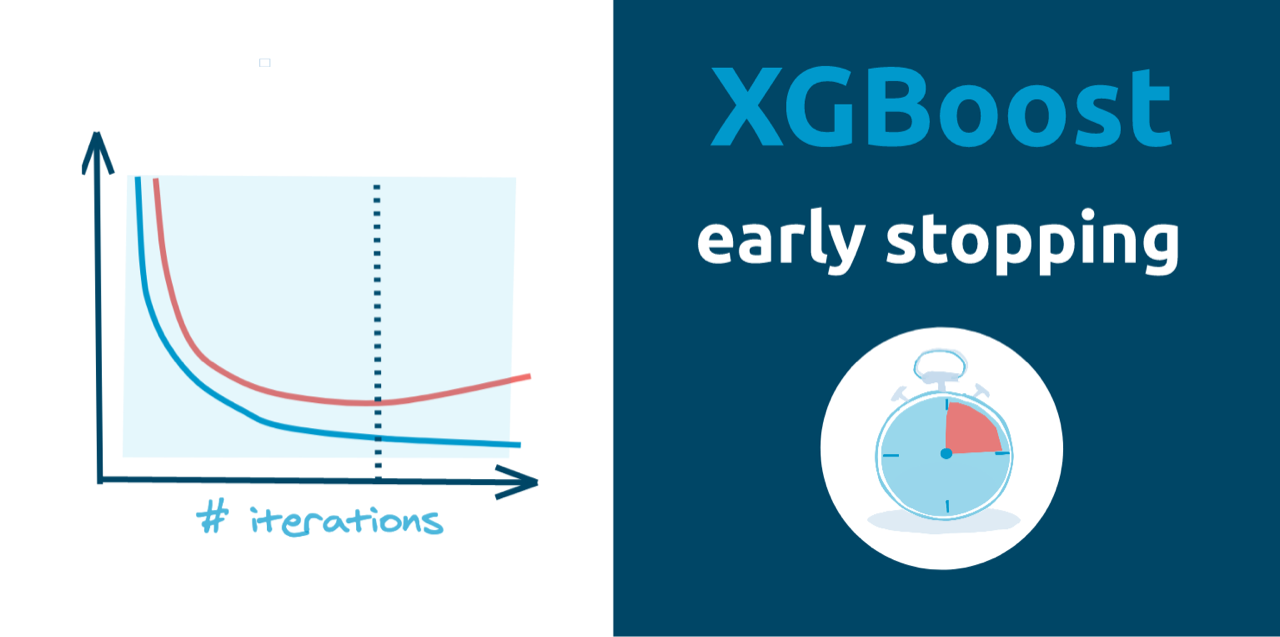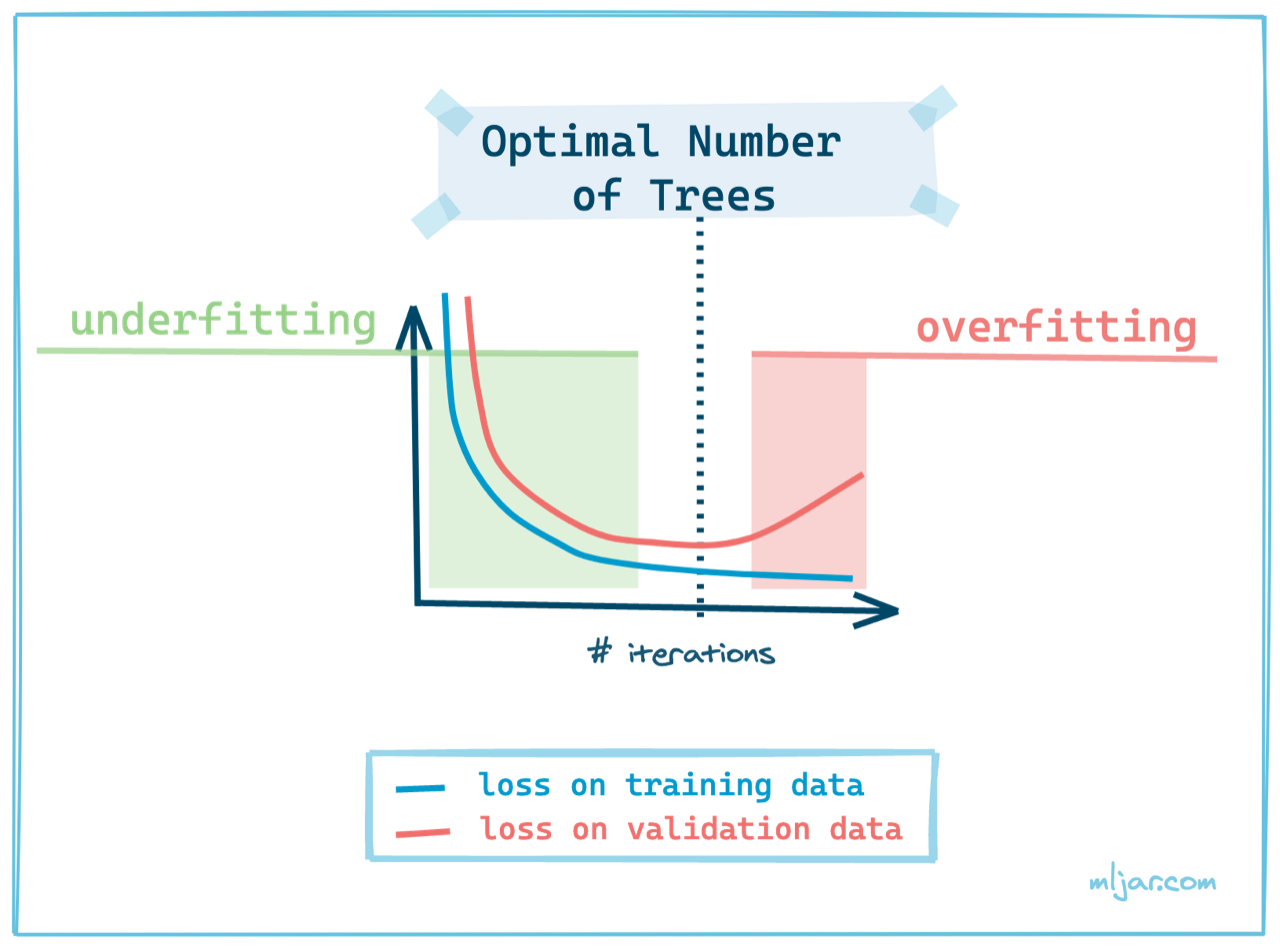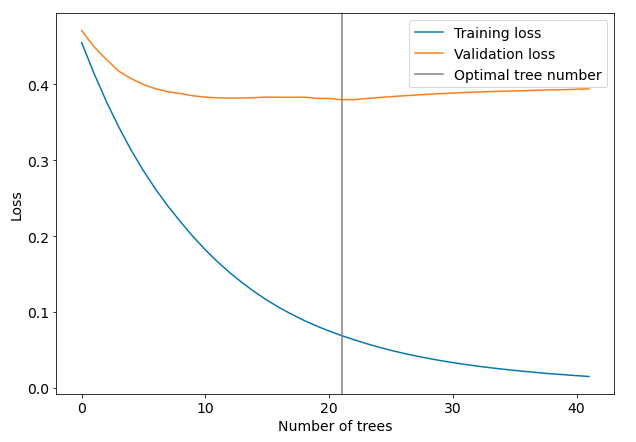Xgboost is a powerful gradient boosting framework that can be used to train Machine Learning models. It is important to select optimal number of trees in the model during the training. Too small number of trees will result in underfitting. On the other hand, too large number of trees will result in overfitting. How to find the optimal number of trees? You can use an early stopping.## Early Stopping

Early stopping is a technique used to stop training when the loss on validation dataset starts increase (in the case of minimizing the loss). That’s why to train a model (any model, not only Xgboost) you need two separate datasets:

• training data for model fitting,
• validation data for loss monitoring and early stopping.

In the Xgboost algorithm, there is an `early_stopping_rounds` parameter for controlling the patience of how many iterations we will wait for the next decrease in the loss value. We need this parameter because the loss values decrease randomly in each iteration. The validation loss can bounce in some range and after few iterations decrease.

How many iterations should early stopping take? I’m using `50` rounds for early stopping (usually with `1000` trees). There are some heuristics that recommend to use `10%` of your total iterations for early stopping, which sounds reasonable.

## Implementation

Let’s show some code example, how to use early stopping during Xgboost training. I’ll be using Xgboost API that is `Scikit-Learn` compatible, because `Learning API` of Xgboost requires manual handling of `best_ntree_limit` for prediction and saving (check this article for details).

#### Create example data

We will create synthetic data set with `sklearn` package and split it to tain and validation samples.

``````# load needed packages
import xgboost as xgb
from sklearn.datasets import make_classification
from sklearn.model_selection import train_test_split
from matplotlib import pyplot as plt

# create dataset
X, y = make_classification(n_samples=100,
n_informative=5,
n_classes=2)
X_train, X_validation, y_train, y_validation = train_test_split(X, y, test_size=0.25)
``````

#### Train Xgboost with Early Stopping

Let’s train the Xgboost model. I set the hyparameters values `max_depth=6`, abd `learning_rate=0.1` to quickly show the overfitting. The `early_stopping_rounds=20` is set for example purposes.

``````model = xgb.XGBRegressor(n_estimators=100, max_depth=6, learning_rate=0.1)
model.fit(X_train, y_train,
eval_set=[(X_train, y_train), (X_validation, y_validtion)],
early_stopping_rounds=20)
``````

The output from model training:

``````> 	validation_0-rmse:0.45517	validation_1-rmse:0.47108
	validation_0-rmse:0.41445	validation_1-rmse:0.44976
	validation_0-rmse:0.37746	validation_1-rmse:0.43300
	validation_0-rmse:0.34385	validation_1-rmse:0.41747
	validation_0-rmse:0.31332	validation_1-rmse:0.40777
	validation_0-rmse:0.28611	validation_1-rmse:0.39987
	validation_0-rmse:0.26140	validation_1-rmse:0.39421
	validation_0-rmse:0.23905	validation_1-rmse:0.39036
	validation_0-rmse:0.21876	validation_1-rmse:0.38798
	validation_0-rmse:0.19948	validation_1-rmse:0.38513
	validation_0-rmse:0.18195	validation_1-rmse:0.38333
	validation_0-rmse:0.16601	validation_1-rmse:0.38237
	validation_0-rmse:0.15152	validation_1-rmse:0.38204
	validation_0-rmse:0.13834	validation_1-rmse:0.38220
	validation_0-rmse:0.12636	validation_1-rmse:0.38271
	validation_0-rmse:0.11546	validation_1-rmse:0.38348
	validation_0-rmse:0.10567	validation_1-rmse:0.38314
	validation_0-rmse:0.09681	validation_1-rmse:0.38311
	validation_0-rmse:0.08878	validation_1-rmse:0.38331
	validation_0-rmse:0.08159	validation_1-rmse:0.38168
	validation_0-rmse:0.07498	validation_1-rmse:0.38157
	validation_0-rmse:0.06904	validation_1-rmse:0.37995
	validation_0-rmse:0.06361	validation_1-rmse:0.38003
	validation_0-rmse:0.05847	validation_1-rmse:0.38143
	validation_0-rmse:0.05380	validation_1-rmse:0.38274
	validation_0-rmse:0.04958	validation_1-rmse:0.38399
	validation_0-rmse:0.04571	validation_1-rmse:0.38509
	validation_0-rmse:0.04219	validation_1-rmse:0.38610
	validation_0-rmse:0.03899	validation_1-rmse:0.38705
	validation_0-rmse:0.03605	validation_1-rmse:0.38795
	validation_0-rmse:0.03340	validation_1-rmse:0.38876
	validation_0-rmse:0.03096	validation_1-rmse:0.38944
	validation_0-rmse:0.02873	validation_1-rmse:0.39003
	validation_0-rmse:0.02668	validation_1-rmse:0.39061
	validation_0-rmse:0.02480	validation_1-rmse:0.39114
	validation_0-rmse:0.02307	validation_1-rmse:0.39153
	validation_0-rmse:0.02148	validation_1-rmse:0.39200
	validation_0-rmse:0.02001	validation_1-rmse:0.39243
	validation_0-rmse:0.01855	validation_1-rmse:0.39308
	validation_0-rmse:0.01744	validation_1-rmse:0.39316
	validation_0-rmse:0.01620	validation_1-rmse:0.39378
	validation_0-rmse:0.01507	validation_1-rmse:0.39421
XGBRegressor(base_score=0.5, booster='gbtree', colsample_bylevel=1,
colsample_bynode=1, colsample_bytree=1, gamma=0, gpu_id=-1,
importance_type='gain', interaction_constraints='',
learning_rate=0.1, max_delta_step=0, max_depth=6,
min_child_weight=1, missing=nan, monotone_constraints='()',
n_estimators=100, n_jobs=36, num_parallel_tree=1, random_state=0,
reg_alpha=0, reg_lambda=1, scale_pos_weight=1, subsample=1,
tree_method='exact', validate_parameters=1, verbosity=None)
``````

The printed loss values can be retrived from the model with `evals_result()` and plotted (picture is worth a thousand words):

``````results = model.evals_result()

plt.figure(figsize=(10,7))
plt.plot(results["validation_0"]["rmse"], label="Training loss")
plt.plot(results["validation_1"]["rmse"], label="Validation loss")
plt.axvline(21, color="gray", label="Optimal tree number")
plt.xlabel("Number of trees")
plt.ylabel("Loss")
plt.legend()
``````As you can see from the plot the optimal number of tree was selected exactly before the loss values started to increase on validation dataset. The training was stopped. We set `n_estimators=100` but only `42` trees were trainined. The optimal numer of trees is 22. We can check the optimal number of trees by printing the `best_ntree_limit` value:

``````model.best_ntree_limit

# results
> 22
``````

To compute the predictions we use `predict()` method, we can pass `ntree_limit` argument, but in the case of `Scikit-Learn` API of Xgboost it is not needed. Both versions `predict()` and `predict(ntree_limit=model.best_ntree_limit)` return the same values:

``````model.predict(X_test)

# result
> array([0.93680346, 0.04154444, 0.9282346 , 0.84900916, 0.0657784 ,
0.92436266, 0.63648796, 0.9468495 , 0.2629984 , 0.9504917 ,
0.04154444, 0.84900916, 0.89390475, 0.9255801 , 0.04154444,
0.07570445, 0.92072046, 0.07966585, 0.9510616 , 0.9365885 ,
0.84900916, 0.07545377, 0.9308903 , 0.9200338 , 0.0657784 ],
dtype=float32)
``````

Set `ntree_limit` in the `predict()` (which means that you select how many trees from model should be used to compute predictions):

``````model.predict(X_test, ntree_limit=model.best_ntree_limit)

# result
> model.predict(X_test, ntree_limit=model.best_ntree_limit)
model.predict(X_test, ntree_limit=model.best_ntree_limit)
array([0.93680346, 0.04154444, 0.9282346 , 0.84900916, 0.0657784 ,
0.92436266, 0.63648796, 0.9468495 , 0.2629984 , 0.9504917 ,
0.04154444, 0.84900916, 0.89390475, 0.9255801 , 0.04154444,
0.07570445, 0.92072046, 0.07966585, 0.9510616 , 0.9365885 ,
0.84900916, 0.07545377, 0.9308903 , 0.9200338 , 0.0657784 ],
dtype=float32)
``````

To save and load model you can simply run:

``````# save
model.save_model("my_xgboost.json")

new_model = xgb.XGBRegressor()
new_model.laod_model("my_xgboost.json")

# check optimal number of trees of loaded model
new_model.best_ntree_limit

# result
> 22
``````

## Conclusions

The `Scikit-Learn` API fo Xgboost python package is really user friendly. You can easily use early stopping technique to prevent overfitting, just set the `early_stopping_rounds` argument during `fit()`. I usually use `50` rounds for early stopping with `1000` trees in the model. I’ve seen in many places recommendation to use about `10%` of total number of trees for early stopping - such recommendation sounds reasonable. With `Scikit-Learn` API of Xgboost you don’t need to worry about selecting optimal number of tree during `predict()` and save and load of the model.### Convert Python Notebooks to Web Apps

We are working on open-source framework Mercury for converting Jupyter Notebooks to interactive Web Applications.### Articles you might find interesing